# Drawing a Scaled Bar Graph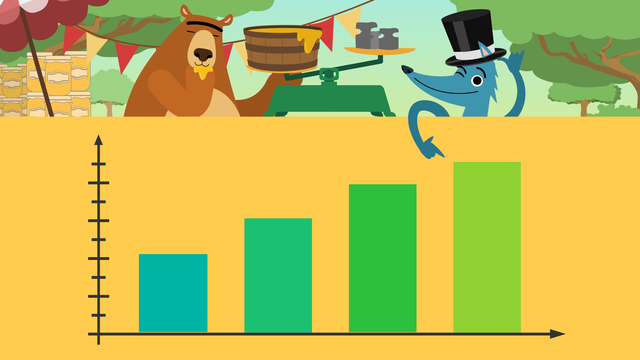Rate this video

Be the first to rate this video!

The authorTeam Digital

## DescriptionDrawing a Scaled Bar Graph

### In this 3rd grade video on scaled bar graphs...

Gus and Nari have started a honey business and need money from the bank to keep it growing. However, the bank will need to see some sales data before lending. In this video, Nari and Gus learn how to organize their information into scaled bar graphs for their investors.

### What is a scaled bar graph?

#### Scaled bar graph definition

A scaled bar graph is a special type of bar graph that uses repeating intervals greater than one to measure the data represented. This is helpful because it allows the graph to show larger ranges of data. Unlike a scaled picture graph, a scaled bar graph does not have a key.

#### Scaled bar graph vs. bar graph

A bar graph is a general term that refers to a type of graph that uses columns to represent sets of information. Whereas, a scaled bar graph is a particular type of bar graph that uses a scaled key to represent and consolidate larger amounts of data.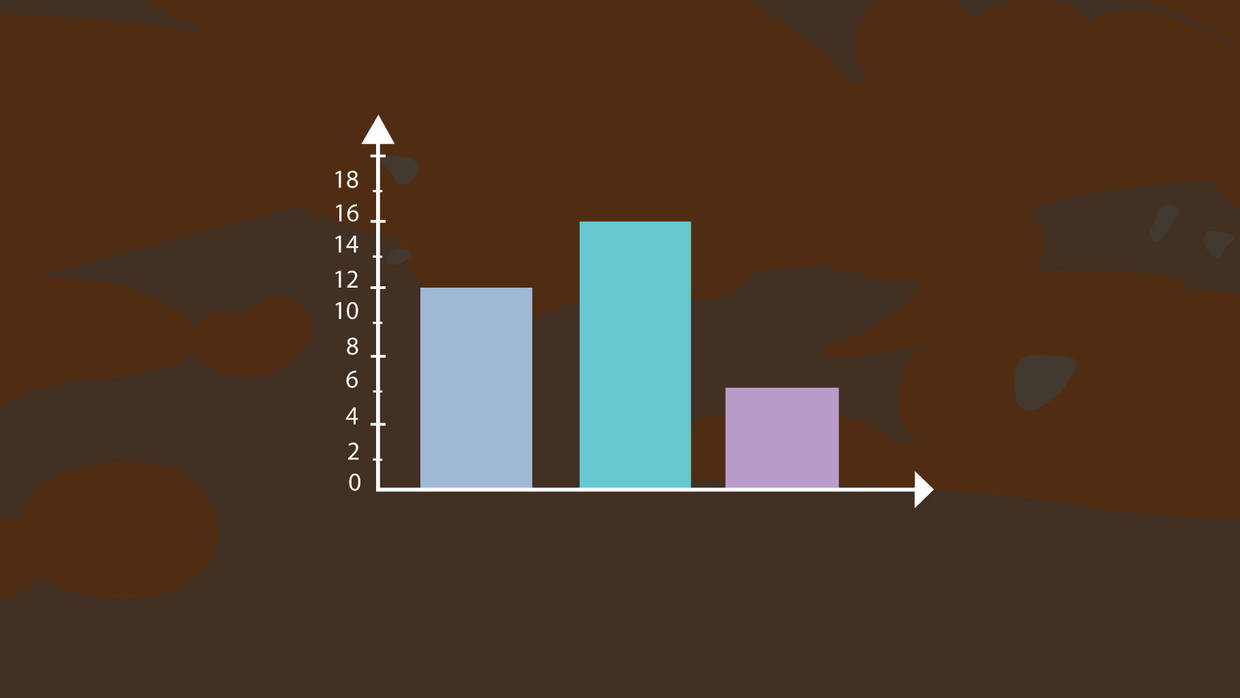### How do you draw a scaled bar graph?

We start drawing a scaled bar graph by identifying our data set. This is the information that we organize and display in the graph. It is important to give this data a name to explain what it represents. This name becomes the title of our graph and will tell our readers what it is about.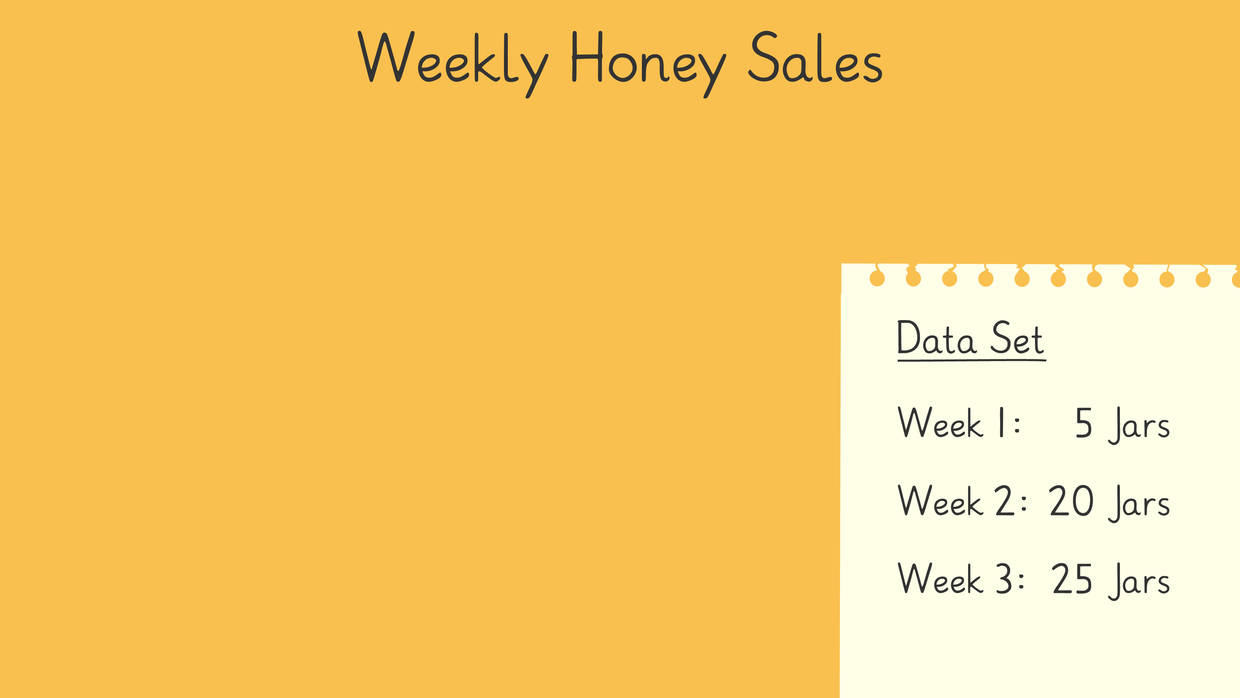Next, we use our data set to begin labelling the axis on our graph. Typically, the x-axis, or horizontal line, represents the data’s categories. Each category is labeled on the x-axis. Then, the entire x-axis is given a name, or subtitle, so readers can identify the types of information.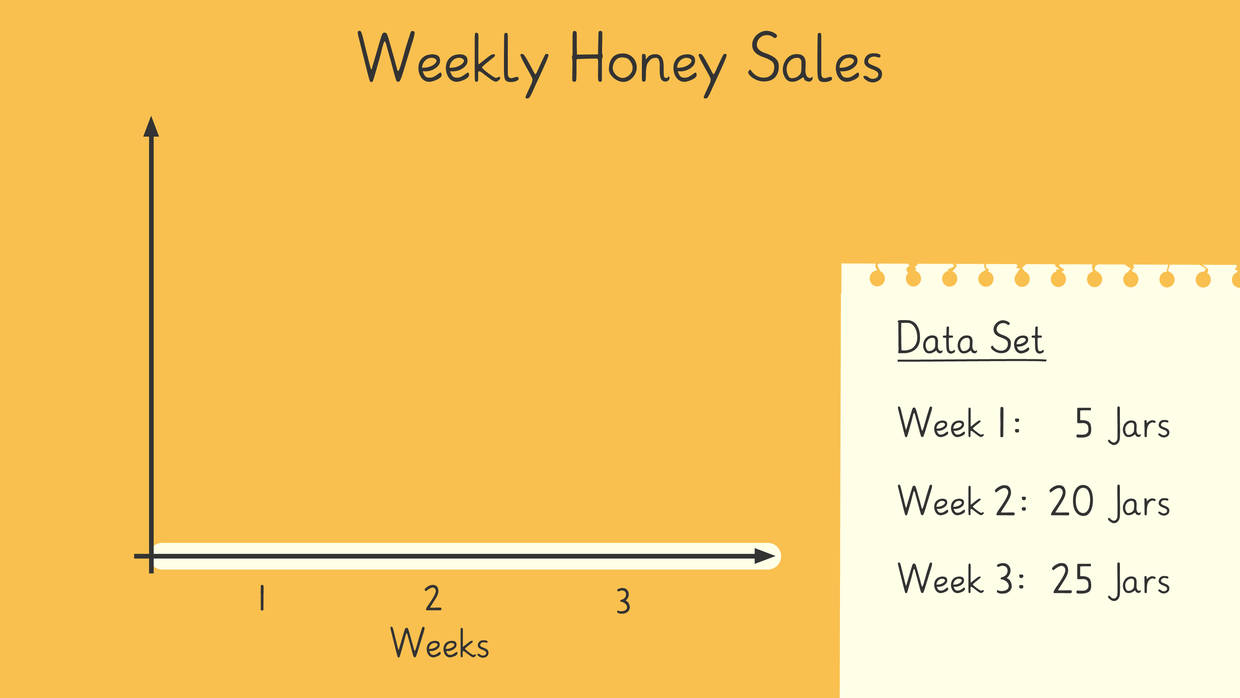Then, we move to the y-axis, or vertical line. Typically, this is where we find the bar graph’s scale. It is the axis that measures the data using a scaled number line. We label this axis with the units it represents (example: dollars, time, total number, etc.). The scale must include all the values in the data set. We determine the graph's range by looking at the smallest and largest numbers in the set. Then, we determine the scale by looking at the other numbers to see if they share a common multiple to count up by. This multiple becomes our scale’s interval. Once the range and interval are determined, we draw the scale on the axis. Scales should begin at zero and go up at the determined interval. Scales should end one interval past the largest number in the data set.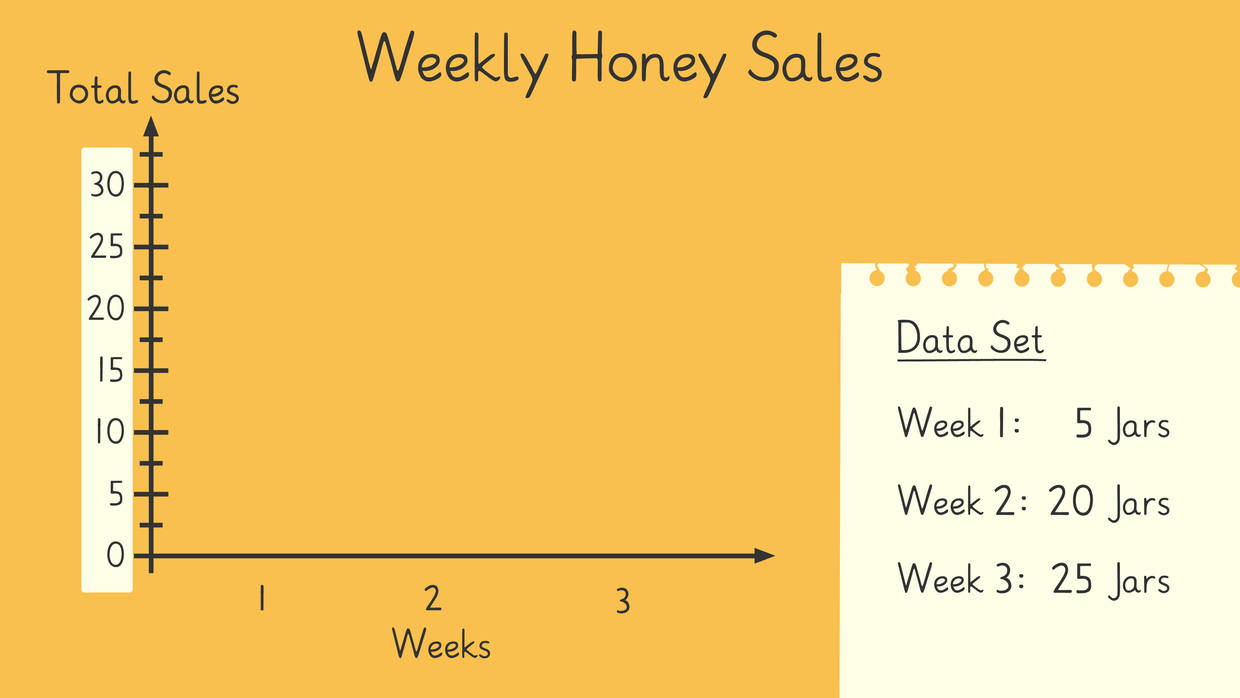Finally, we plot the data. Carefully draw each bar by finding the data in the set. Match it with the same category on the x-axis and draw your bar from zero to the total number. Repeat this process until all the data has been plotted.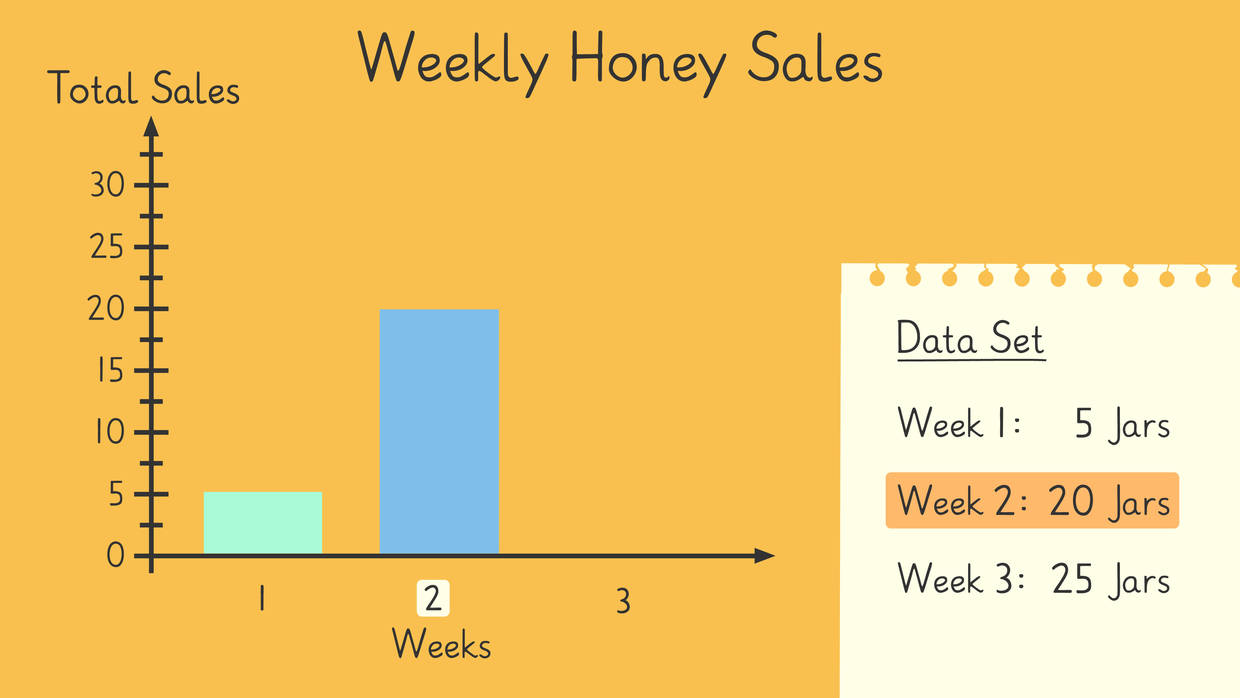### Determine the scale on a bar graph

Learn how to figure out the scale for a bar graph below.

#### How would you choose a scale on a bar graph?

To determine a bar graph's scale interval, find the smallest and largest numbers in the data set to define the range. Then, look at the other numbers in the set to see if they share a common multiple to count up by. This common number becomes the scale’s interval. Once the range and interval are determined, draw the scale on the axis. Scales should begin at zero and go up at the determined interval. End the scale one interval past the largest number in the data set.

### What does a scaled bar graph look like?

Below are scaled bar graph examples.

An example of a general scaled bar graph.A scaled bar graph with intervals of five.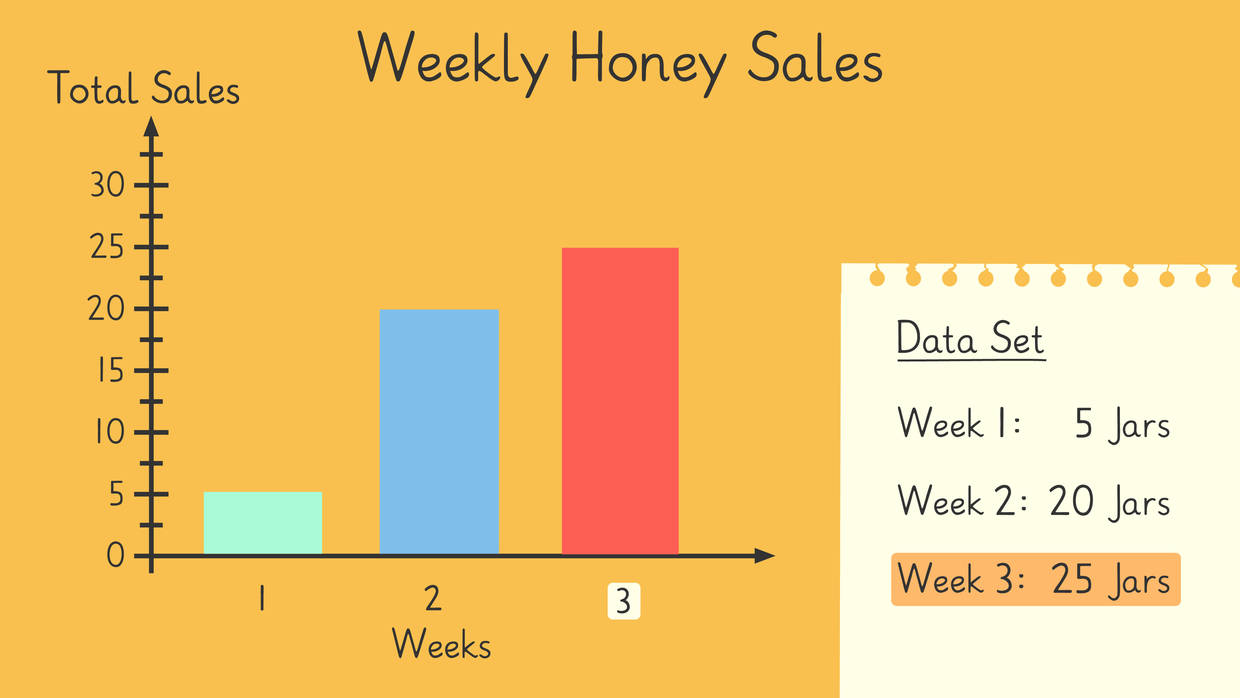A scaled bar graph with intervals of ten.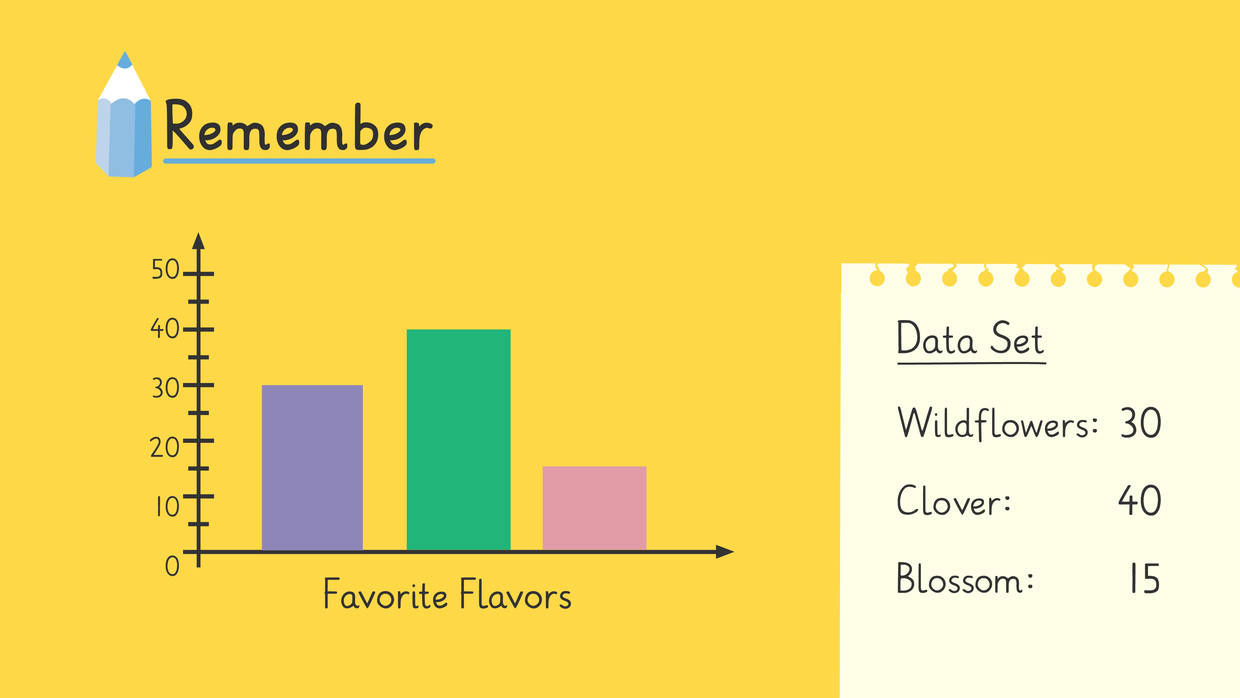### Summary of Steps: Making a Scaled Bar Graph

• When drawing a scaled bar graph, first, title your graph by naming the data set.

• Next, label your categories on the x- and y-axes.

• Then, create the scale for the y-axis by including the whole data set and looking for a common multiple to use as the interval.

• Finally, plot the data on the graph by drawing bars.

Have you practiced yet? On this website, you can also find scaled bar graph worksheets and exercises.

### TranscriptDrawing a Scaled Bar Graph

Gus and Nari have started a honey business and need money from the bank to keep it growing, but the bank asked for their honey sales data before lending money. Let's help Nari and Gus make scaled bar graphs to show their data! Drawing a Scaled Bar Graph Remember! A bar graph is a type of graph that uses columns to represent sets of information! In a scaled bar graph, the scale that measures the bars uses repeating intervals greater than one. Our first graph will show how many honey jars Nari and Gus sell each week. First, we'll represent the data by titling the graph weekly honey sales. Next, we will look at our data set - this is the information we know about the sales each week. We'll use the data set to start drawing our graph. The x-axis, or horizontal line, represents our weekly data so let's write one, two, three like the paper and label it "Weeks". The y-axis, or vertical line, measures the amount of jars sold so we'll draw a scale that includes all of their sales. We determine the graph's scale by looking at the smallest and largest numbers to include the entire data set. Then, we look at the other numbers to see if they share a common multiple to count up by. This data set shares the multiple five and includes the numbers five through twenty-five. Our scale should begin at zero and go up at intervals of five until we reach thirty, one interval past our largest number. It's time to plot our data on the graph. During week one, Nari and Gus sold five jars. Let's draw a bar from zero to five. Nari and Gus sold twenty jars in week two, so we draw a bar from zero to twenty above 'week two'. What do we draw above 'week three'? Nari and Gus sold 25 jars, that means we need to draw a bar from zero to twenty five above week three. Now that we've completed the first graph, let's make one more. Our second graph needs to show which honey is the most popular. We begin by giving our graph a title. Let's call it favorite flavors, so we know what the data represents. To start our graph, what do we write on the x-axis? This data set includes the most popular flavors...Wildflower... Clover...Blossom...so we'll write the most popular flavors on the x-axis. What do we draw on the y-axis? We draw the scale that includes how many customers like each flavor. Remember, we determine the scale by looking at the smallest and largest numbers to include the entire data set. Then, we look at the other numbers to see if they share a common multiple to count up by. Most of this data set shares the multiple ten and includes the numbers fifteen through forty. Our scale should begin at zero and go up at intervals of ten until we reach fifty, one interval past our largest number. Let's plot our data on the graph. Nari and Gus have thirty customers who like Wildflower so here we draw a bar that begins at zero and reaches thirty. What will we draw above the word Clover? We will draw a bar that begins at zero and reaches forty because they have forty customers who prefer Clover. If they have fifteen customers who like Blossom most, what do we draw there? We draw a bar that only reaches halfway between ten and twenty on the scale because fifteen is halfway between them!" Next, label your categories on the x and y axis. Then, create a scale for your Y-axis by including the whole data set and looking for a common multiple to use as the interval. And, finally, plot your data on the graph by drawing your bars. "THIS is just the kind of strategic purchase we needed to get this honey company off the ground!"